ip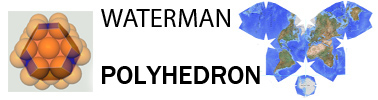3 charts related ionization energies conversion
over 100 physical constants in my inter-relationship fundamental constant chart

Why I started to question the fundamental physical constant values at NIST...
.
I noticed a mahematical pattern that extended past/ was more comprehensive than some measured values regarding the energies of electrons around the nucleus. A constant emerged with a value of 13.62194; which they simply called A, by NIST. This mathematical value I had gleaned, winds up being extremely close to another measured value of NIST's...called the Rydberg constant.
 ionization energies...where the value for A = Rydberg constant at 13.62164 versus NIST at 13.60569253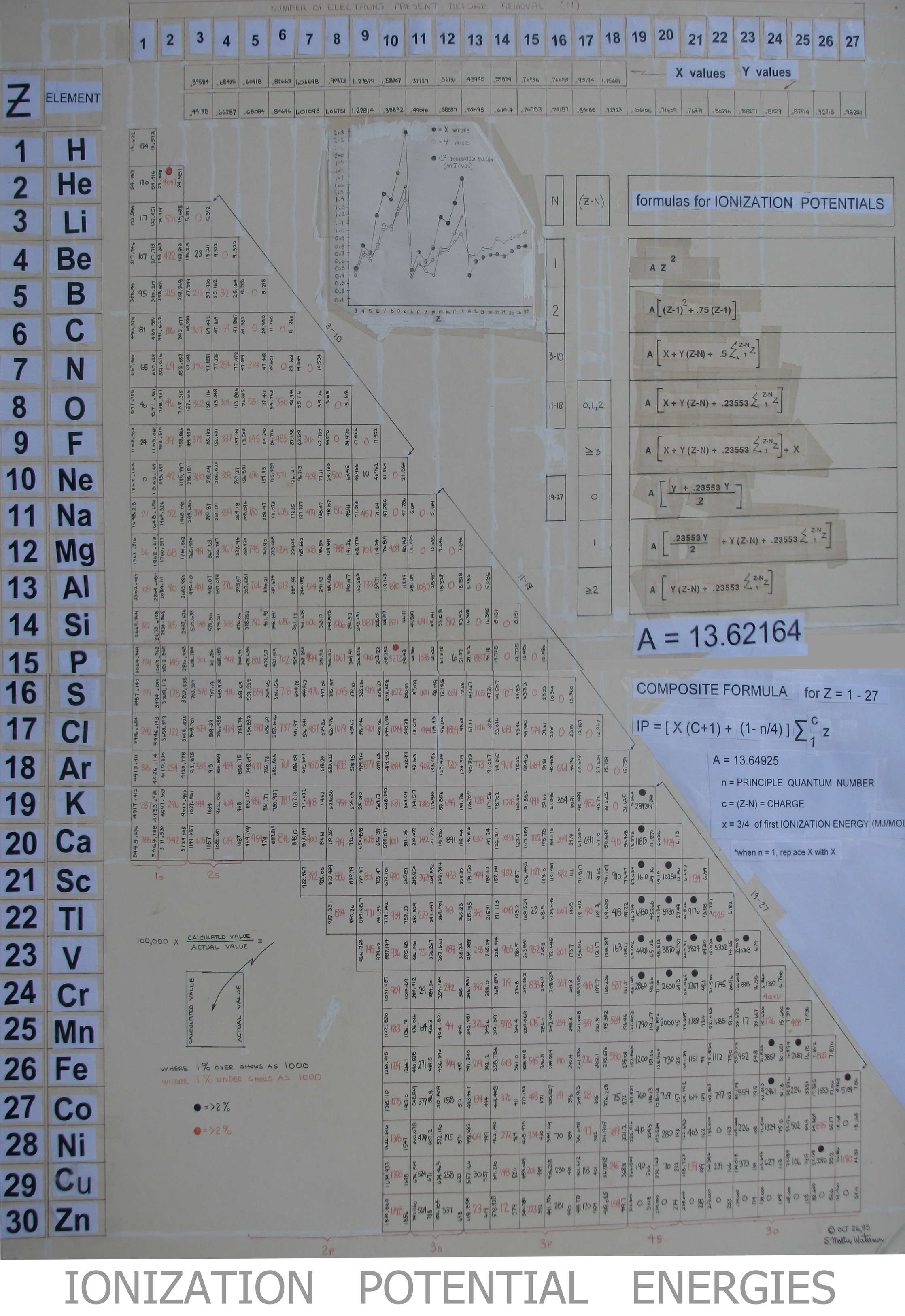From my mathematical studies of clusters of fcc packing, the cluster quantities where compared to total ionization energy. One number popped out, and when questioned, the head professer re constants at McGill University, said he had never heard of this particular value. Physics calls it the inverse-meter relationship electron volt relatoionship. Chemists however, call it the ionization conversion factor. When I took, my Rydberg constant value and divided by my ionization factor, this numerically was quite close to another constant value; Ryberg at infinty NIST = 10,973,731.568589 versus 1.09662271123215 ionization energies values seen as three component values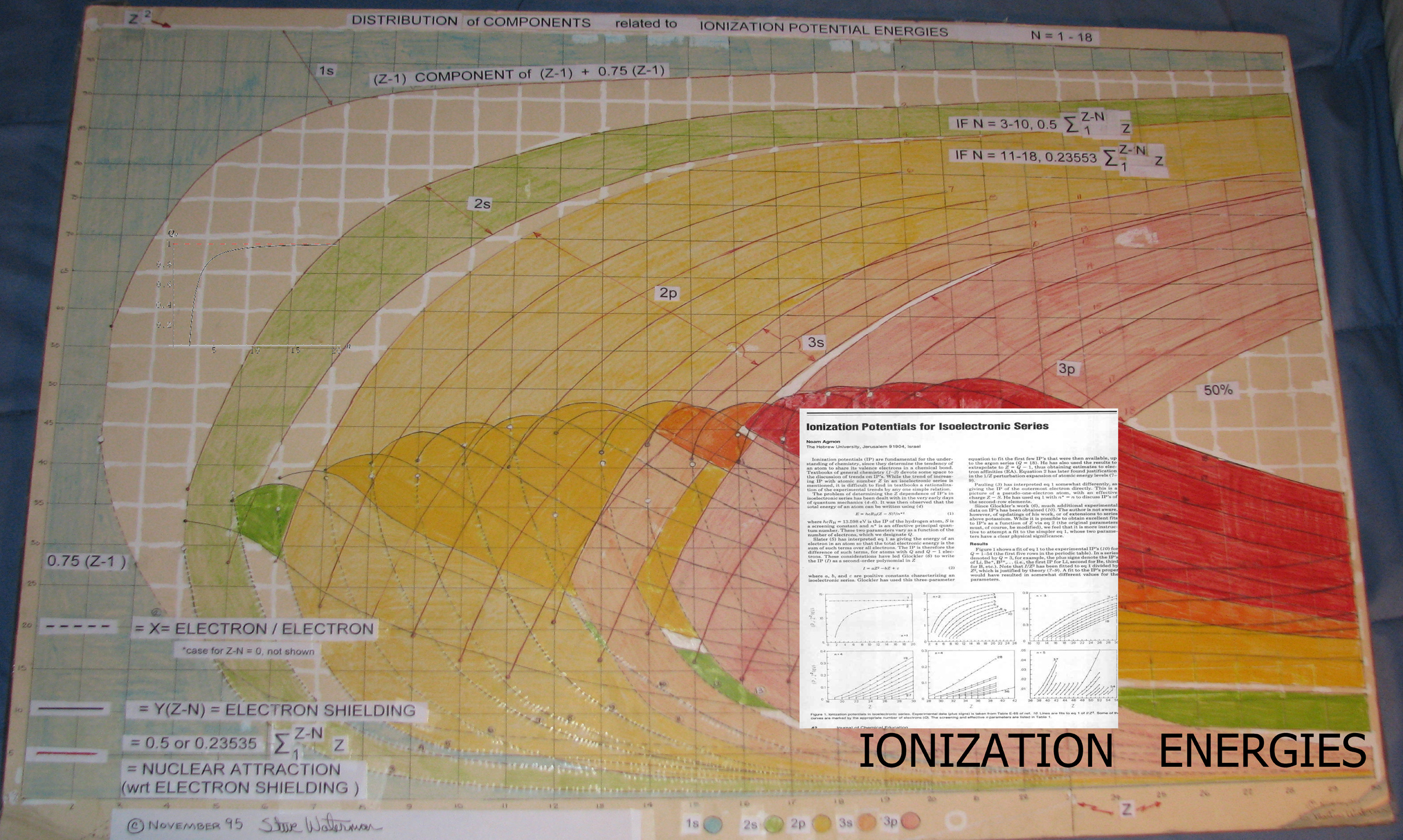sphere counts and the ionization converstion factor 12,398. versus NIST 1.239841930.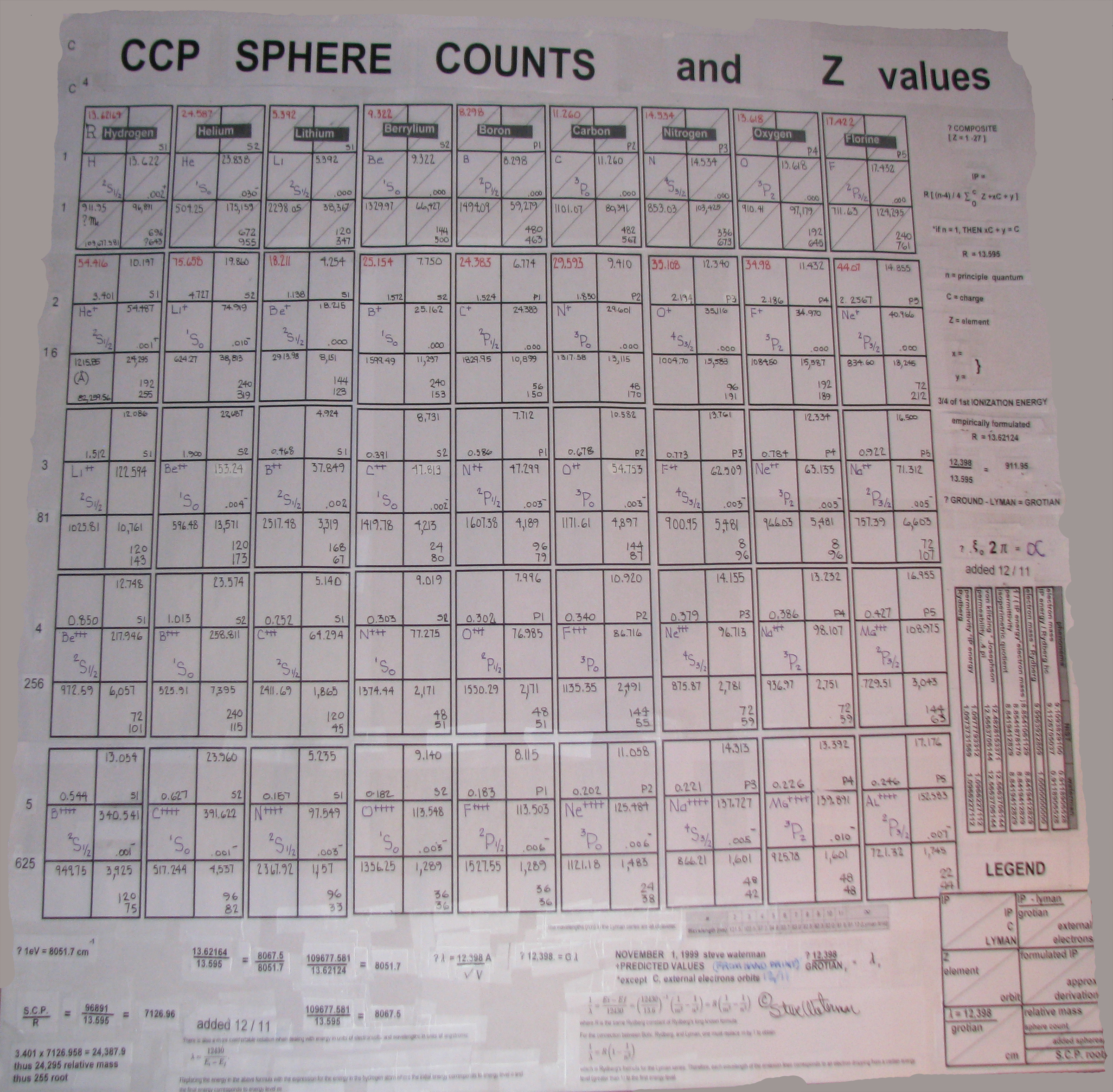That made me want to see if I could find the relationship of other constants, for instance, what is called the permittivity of as vacuum. Long, long story, cut real real short. Various mathematical techniques were employed to attempt to further investigate possibe values. Initially, a small list of major constant values were placed into excel and a cross-multipying of all values was done. Then all results ( carrying the factor names ) were placed into a numerical sequence by A times B results. Mutiplication results were confined to 1 to less than 10.      Later, it was noticed that many values had factors of 2 or 3 or π. A scheme was manifested to throw constant values into the excel cross-mutiplication restricted to low integer exponents of 2 x 3 x π also allowing 2 or 3 to have 1/2 integer exponents. My brother Don, wrote a special sub-routine into several dedicated programs that took these exponents as factors of 2 x 3 x π, and gave back, a 15 place decimal value...forced to between 1 and less than 10 x 10 to the n. All known mathematical constants were tried. Fast forward a decade-ish, and thousands of attempts to make a like chart...this one finally worked....being spaced into a grid-like format.   over 100 physical constants in my inter-relationship chart ( each physical constant only used once )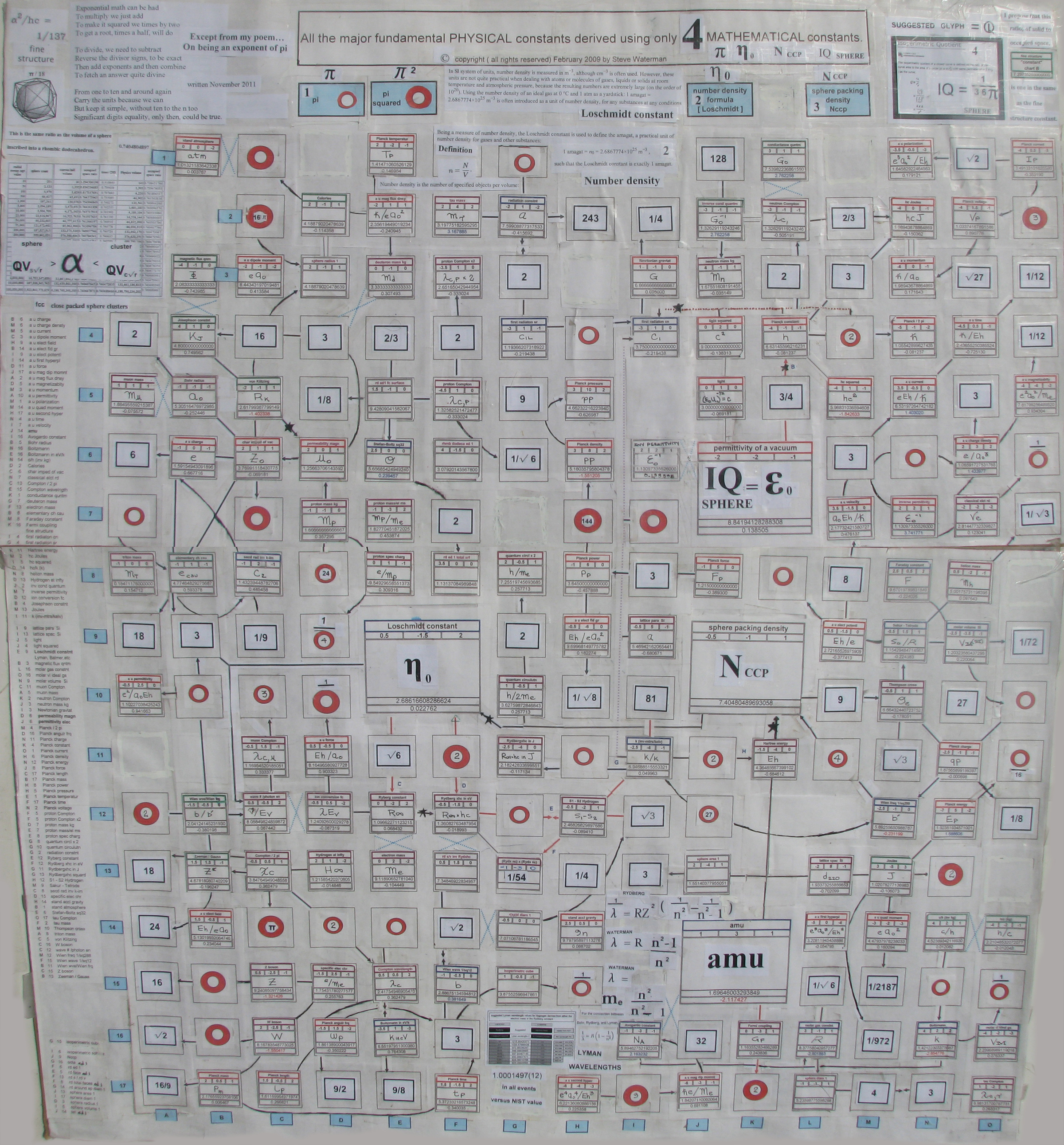constants poem.....deriving all the major fundamental constant values with only a handful of math constants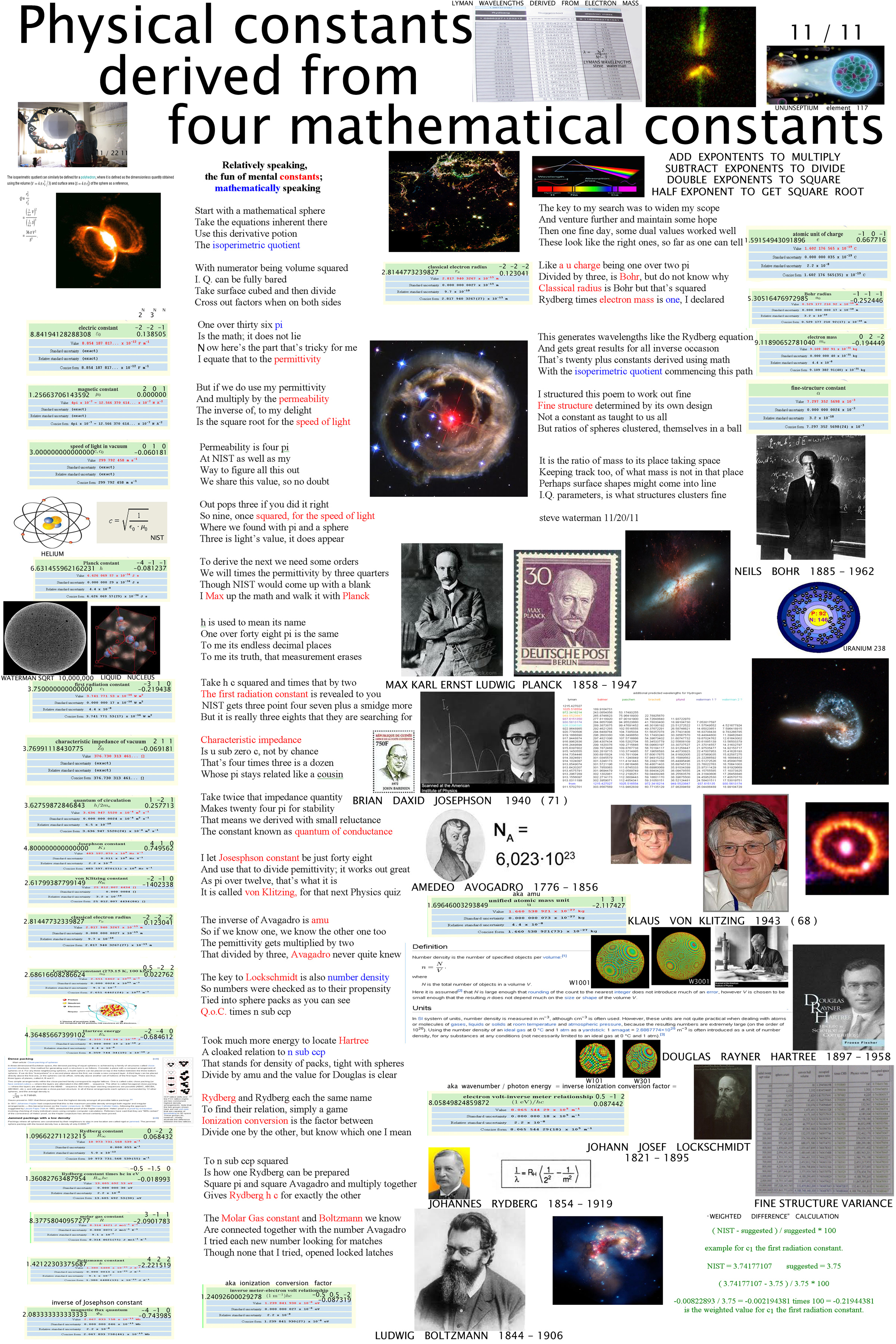back